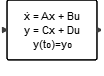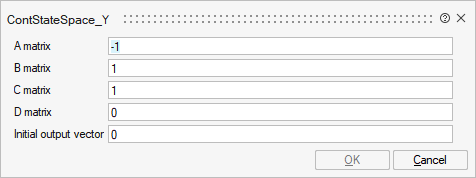# ContStateSpace_Y

This block implements a continuous-time, linear, state-space system specified by the A, B, C, D matrices. The state vector is computed in such a way that the requested initial output is obtained at the initial time.## Description

This block implements a continuous-time, linear, state-space system specified by the A, B, C, D matrices. The state vector is computed in such a way that the requested initial output is obtained at the initial time.

## ParametersNameLabelDescriptionData TypeValid Values

A

A matrix

real nxn matrix where n is the number of states, default value: 1.

Matrix

B

B matrix

real nxm matrix where n is the number of states and m is the number of inputs, default value: 1. B=[ ] if block has no input.

Matrix

C

C matrix

real rxn matrix, default value: 1. r is the number of outputs and n is the number of states.

Matrix

D

D matrix

real rxm matrix where r is the number of outputs and m is the number of inputs, default value: 1.

Matrix

y0

Initial output vector

vector of size r.

Vector

## Ports

NameTypeDescriptionIO TypeNumber

Port 1

explicit

output

1

Port 2

explicit

input

1

NameValueDescription

always active

yes

direct-feedthrough

no, except when D not zero

zero-crossing

no

mode

no

continuous-time state

yes, except when n is zero

discrete-time state

no